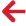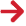﻿ PipeLay > Software Components & Operation > Analysis & Postprocessing > Analysis Component > Fatigue Tab > Fatigue - Normal Lay - Outputs

# Fatigue - Normal Lay - OutputsThe outputs from a Normal Lay fatigue analysis are as follows:

Total Fatigue Damage. The total fatigue damage occurring during installation.

Standby Time. The maximum time the suspended pipe can be held stationary without exceeding the Allowable Fatigue Damage.

Maximum Nodal Fatigue Damage Rate. The maximum fatigue damage rate for all nodes along the pipeline.

Maximum Fatigue Damage Rate at TDP. The maximum fatigue damage rate for the touchdown point.

Plot of Variation of Damage Rate along Pipe. Nine plots are generated in total - one plot for each of the eight points on the pipeline circumference, and a ninth plot showing the maximum of all eight values at each node.

Plot of Maximum Rate of Change in Restoring Force Statistics. Six graphs are presented on a single plot; one each for the mean and standard deviation of axial force, y-bending moment and z-bending moment.

The final output requires some elaboration. While the dynamic random sea analysis is in progress, the mean value and standard deviation of axial force, y-bending moment and z-bending moment at each node is continuously calculated. For each of these six parameters, a rate of change in value from the previous solution time is then computed. From the values at all nodes, the maximum rate of change for each of the six parameters is selected and stored for subsequent plotting. These are plotted as a function of time. The objective is to provide you with some measure to check if the random sea analysis duration is of sufficient length. If it is, the rate of change in the statistics will reduce continuously (the statistics will reach steady state). Note that in addition to this output being available after the fatigue analysis completes, it is also available during runtime and may be viewed by clicking on the Progress tab of the Analysis component. This allows you to stop an analysis before the specified End Time if statistics have reached steady state. Note that this output only applies to the Full fatigue Analysis Option.

There are additional optional outputs if you set to Yes; they are as follows:

Fatigue_hist.csv. Contains the stress histogram data for 8 circumferential points at each node. This can be viewed by clicking the Open Histogram Output button on the Results tab.

Normal Lay Weld Stress Histogram Data. This is the accumulated weld stress histogram data for 8 circumferential points as well as a governing stress histogram. This data can be viewed in the fatigue.out file.

Plots of Normal Lay Weld Stress Histograms. Nine plots are generated in total – one accumulated weld stress histogram for each of the 8 circumferential points of the pipeline, and a ninth plot  containing the governing weld stress histogram which contains the maximum of the eight values for each bin.